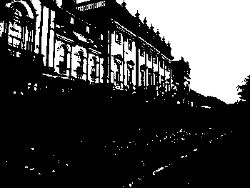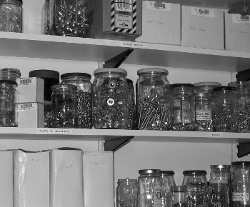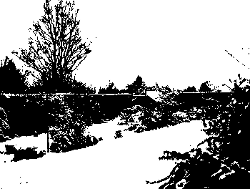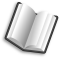# The Lab Book Pages

An online collection of electronics information

Dr. Andrew Greensted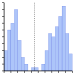# Otsu Thresholding

Converting a greyscale image to monochrome is a common image processing task. Otsu's method, named after its inventor Nobuyuki Otsu, is one of many binarization algorithms. This page describes how the algorithm works and provides a Java implementation, which can be easily ported to other languages. If you are in a hurry, jump to the code.

Many thanks to Eric Moyer who spotted a potential overflow when two integers are multiplied in the variance calculation. The example code has been updated with the integers cast to floats during the calculation.

## Otsu Thresholding Explained

Otsu's thresholding method involves iterating through all the possible threshold values and calculating a measure of spread for the pixel levels each side of the threshold, i.e. the pixels that either fall in foreground or background. The aim is to find the threshold value where the sum of foreground and background spreads is at its minimum.

The algorithm will be demonstrated using the simple 6x6 image shown below. The histogram for the image is shown next to it. To simplify the explanation, only 6 greyscale levels are used.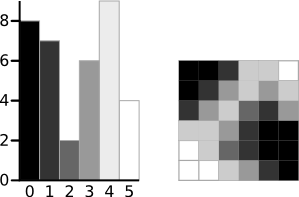A 6-level greyscale image and its histogram

The calculations for finding the foreground and background variances (the measure of spread) for a single threshold are now shown. In this case the threshold value is 3.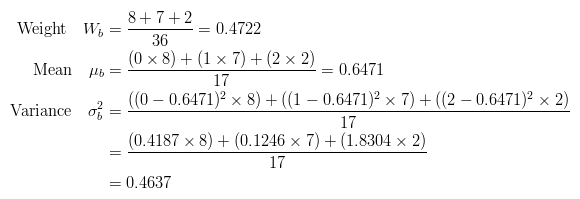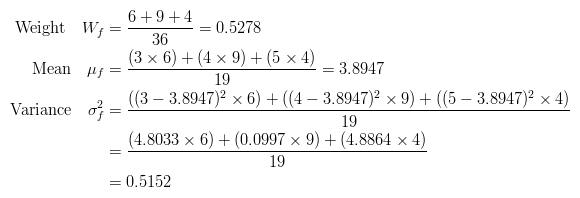The next step is to calculate the 'Within-Class Variance'. This is simply the sum of the two variances multiplied by their associated weights.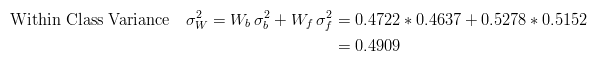This final value is the 'sum of weighted variances' for the threshold value 3. This same calculation needs to be performed for all the possible threshold values 0 to 5. The table below shows the results for these calculations. The highlighted column shows the values for the threshold calculated above.

 Threshold `T=0` `T=1` `T=2` `T=3` `T=4` `T=5`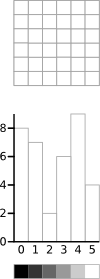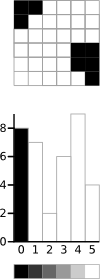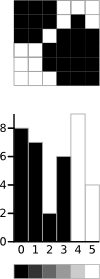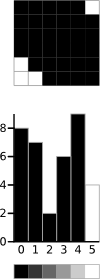Weight, Background `Wb = 0` `Wb = 0.222` `Wb = 0.4167` `Wb = 0.4722` `Wb = 0.6389` `Wb = 0.8889` Mean, Background `Mb = 0` `Mb = 0` `Mb = 0.4667` `Mb = 0.6471` `Mb = 1.2609` `Mb = 2.0313` Variance, Background `σ2b = 0` `σ2b = 0` `σ2b = 0.2489` `σ2b = 0.4637` `σ2b = 1.4102` `σ2b = 2.5303` Weight, Foreground `Wf = 1` `Wf = 0.7778` `Wf = 0.5833` `Wf = 0.5278` `Wf = 0.3611` `Wf = 0.1111` Mean, Foreground `Mf = 2.3611` `Mf = 3.0357` `Mf = 3.7143` `Mf = 3.8947` `Mf = 4.3077` `Mf = 5.000` Variance, Foreground `σ2f = 3.1196` `σ2f = 1.9639` `σ2f = 0.7755` `σ2f = 0.5152` `σ2f = 0.2130` `σ2f = 0` Within Class Variance `σ2W = 3.1196` `σ2W = 1.5268` `σ2W = 0.5561` `σ2W = 0.4909` `σ2W = 0.9779` `σ2W = 2.2491`

It can be seen that for the threshold equal to 3, as well as being used for the example, also has the lowest sum of weighted variances. Therefore, this is the final selected threshold. All pixels with a level less than 3 are background, all those with a level equal to or greater than 3 are foreground. As the images in the table show, this threshold works well.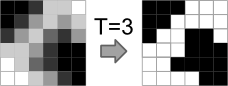Result of Otsu's Method

This approach for calculating Otsu's threshold is useful for explaining the theory, but it is computationally intensive, especially if you have a full 8-bit greyscale. The next section shows a faster method of performing the calculations which is much more appropriate for implementations.

## A Faster Approach

By a bit of manipulation, you can calculate what is called the between class variance, which is far quicker to calculate. Luckily, the threshold with the maximum between class variance also has the minimum within class variance. So it can also be used for finding the best threshold and therefore due to being simpler is a much better approach to use.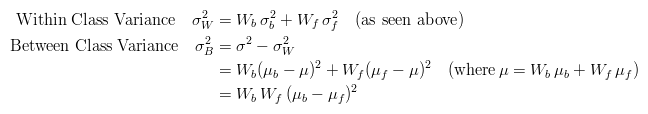The table below shows the different variances for each threshold value.

 Threshold `T=0` `T=1` `T=2` `T=3` `T=4` `T=5` Within Class Variance `σ2W = 3.1196` `σ2W = 1.5268` `σ2W = 0.5561` `σ2W = 0.4909` `σ2W = 0.9779` `σ2W = 2.2491` Between Class Variance `σ2B = 0` `σ2B = 1.5928` `σ2B = 2.5635` `σ2B = 2.6287` `σ2B = 2.1417` `σ2B = 0.8705`

## Java Implementation

A simple demo program that uses the Otsu threshold is linked to below.

Otsu Threshold Demo

The important part of the algorithm is shown here. The input is an array of bytes, `srcData` that stores the greyscale image.

File Excerpt: `OtsuThresholder.java`
```// Calculate histogram
int ptr = 0;
while (ptr < srcData.length) {
int h = 0xFF & srcData[ptr];
histData[h] ++;
ptr ++;
}

// Total number of pixels
int total = srcData.length;

float sum = 0;
for (int t=0 ; t<256 ; t++) sum += t * histData[t];

float sumB = 0;
int wB = 0;
int wF = 0;

float varMax = 0;
threshold = 0;

for (int t=0 ; t<256 ; t++) {
wB += histData[t];               // Weight Background
if (wB == 0) continue;

wF = total - wB;                 // Weight Foreground
if (wF == 0) break;

sumB += (float) (t * histData[t]);

float mB = sumB / wB;            // Mean Background
float mF = (sum - sumB) / wF;    // Mean Foreground

// Calculate Between Class Variance
float varBetween = (float)wB * (float)wF * (mB - mF) * (mB - mF);

// Check if new maximum found
if (varBetween > varMax) {
varMax = varBetween;
threshold = t;
}
}
```

## Examples

Here are a number of examples of the Otsu Method in use. It works well with images that have a bi-modal histogram (those with two distinct regions).

Greyscale Image Binary Image Histogram